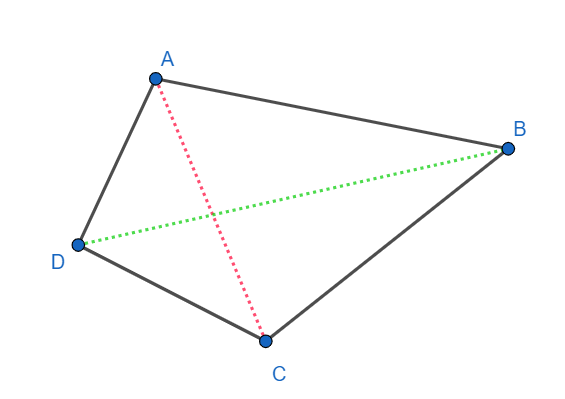QUESTION

# How many diagonals does a convex quadrilateral have?[a] 1[b] 2[c] 3[d] 4

Hint: Recall that a diagonal is a line segment formed by two points of a polygon such that the points are not connected by the side of the polygon. Use the definition of a convex polygon and think how many diagonals can a convex quadrilateral have.

We know that a diagonal of a polygon is a line segment formed by joining two points of the polygon which are not connected by the side of the polygon.
A convex polygon is a polygon having the following property:
If any two points A and B are inside the polygon, then the whole line segment AB is inside the polygon. Hence a convex quadrilateral has all angles smaller than $180{}^\circ$.ABCD is an example of a convex quadrilateral. It is clear from the picture that only the pairs of point (A, C) and (B, D) are not connected by a side.
Hence ABCD has two diagonals AC and BD.
Hence a convex quadrilateral has 2 diagonals.
Hence option [b] is correct.

Note: Alternatively, we know that the number of diagonals in a polygon of n sides is given by
$\dfrac{\left( n \right)\left( n-3 \right)}{2}$.
Put n= 4, we get
The number of diagonals in a quadrilateral $=\dfrac{4\left( 1 \right)}{2}=2$
Hence option [b] is correct.